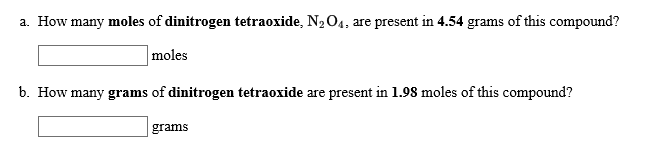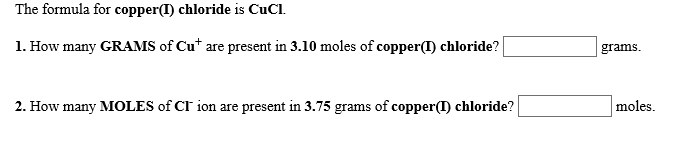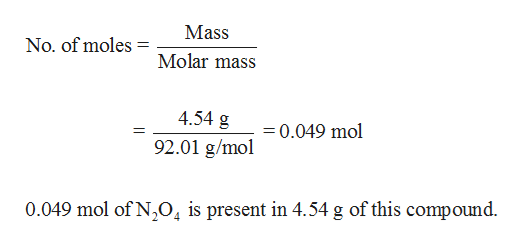# a. How many moles of dinitrogen tetraoxide, N2 O4. are present in 4.54 grams of this compound?molesb. How many grams of dinitrogen tetraoxide are present in 1.98 moles of this compound?grams The formula for copper(I) chloride is CuCl1. How many GRAMS of Cu* are present in 3.10 moles of copper(T) chloride?gramsmoles2. How many MOLES of CI ion are present in 3.75 grams of copper(I) chloride?

Question
2 viewshelp_outlineImage Transcriptionclosea. How many moles of dinitrogen tetraoxide, N2 O4. are present in 4.54 grams of this compound? moles b. How many grams of dinitrogen tetraoxide are present in 1.98 moles of this compound? grams fullscreenhelp_outlineImage TranscriptioncloseThe formula for copper(I) chloride is CuCl 1. How many GRAMS of Cu* are present in 3.10 moles of copper(T) chloride? grams moles 2. How many MOLES of CI ion are present in 3.75 grams of copper(I) chloride? fullscreen
check_circle

Step 1

Hey, since there are multiple questions posted, we will answer first question. If you want any specific question to be answered then please submit that question only or specify the question number in your message.

Step 2

(a)

Number of moles of dinitrogen tetraox...help_outlineImage TranscriptioncloseMass No. of moles Molar mass 4.54 g 0.049 mol 92.01 g/mol 0.049 mol of N,04 is present in 4.54 g of this compound. fullscreen

### Want to see the full answer?

See Solution

#### Want to see this answer and more?

Solutions are written by subject experts who are available 24/7. Questions are typically answered within 1 hour.*

See Solution
*Response times may vary by subject and question.
Tagged in

### General Chemistry